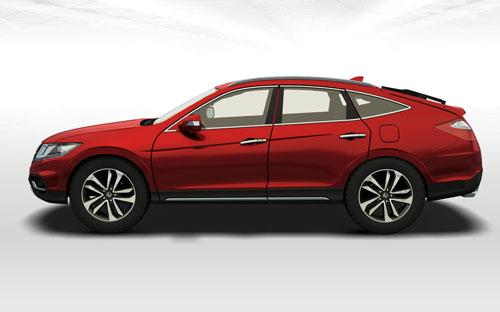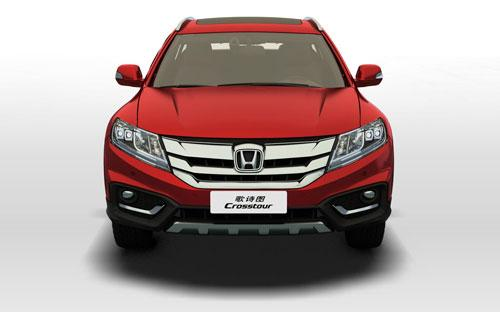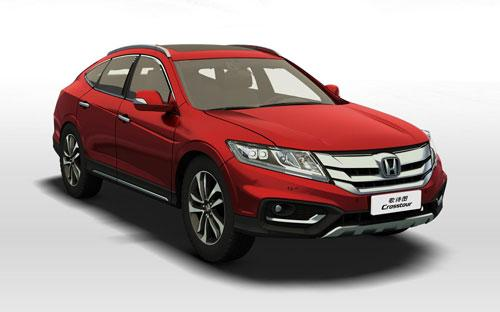广汽本田 歌诗图5 种颜色可选2016款最低售价：25.98 万元起

4995(mm)1900(mm)1560(mm)配置亮点：
• 胎压监测装置

• ISOFIX儿童座椅接口

• 车身稳定控制(ESC/ESP/DSC等)

• 电动天窗

• 定速巡航

• 后倒车雷达

• 真皮座椅

• GPS导航系统

• 氙气大灯

• 后视镜加热

• 提交
2016款 2.4L 豪华版 (139张)

广汽本田 歌诗图 绕车实拍• 广汽本田 歌诗图 在售车型

排量 车型 厂商指导价 本地最低报价 购车工具
2.4L
2.4L 豪华版 5挡自动
25.98万
26.58万
3.0L AWD尊贵版 6挡手自一体
32.98万
3.0L AWD尊贵导航版 6挡手自一体
33.58万
3.0L
2.4L 豪华版 5挡自动
25.98万
26.58万
3.0L AWD尊贵版 6挡手自一体
32.98万
3.0L AWD尊贵导航版 6挡手自一体
33.58万

广汽本田 歌诗图 经销商

查看更多 >>

广汽本田 歌诗图 动力加速

歌诗图 0-100公里加速时间分布在 0.0-秒 属于 超跑级

动力级别 加速时间 车型

广汽本田 歌诗图 视频

广汽本田 歌诗图 新闻资讯

本田2016款歌诗图上市 售价25.98-33.58万

国产新车 超过9190次关注

广汽本田正式宣布，2016款歌诗图正式上市，在外观方面与老款车型保持一致，内饰上增加棕色配色，继续搭载2.0L和3.0L两种动力共计四款车型，其售价区间为25.98-33.58万元。

偷得浮生半日闲 体验本田歌诗图2.4L

评测 超过3263次关注

北京的冬日让人不太提得起精神，特别是当季连绵不断的阴沉雾霾更是令人扫兴。所以当多日未见的午后温暖阳光再次洒向这个其实不想这么奔波忙碌的可爱城市的时候，我...

歌诗图购车手册 推荐2.4L自动豪华导航版

导购 超过3508次关注

2010年年底的时候，广汽本田正式推出了了第一款跨界车歌诗图，仅搭载一款3.5LV6发动机，共有2个配置，售价接近40万。歌诗图搭载的这款全球十佳发动机加上自身新颖的...

本田在美召回Crosstour 前气囊存安全隐患

新闻 超过3857次关注

据海外媒体报道，超过3,300辆的2010款本田Crosstour在美国市场进行召回，原因是前排乘客座席气囊存在潜在的安全问题。

2012款歌诗图购车优惠2.6万元 少量现车

新闻 超过2695次关注

日前，编辑从广汽本田汽车国鑫特约销售服务店了解到，目前歌诗图店内少量现车供应，售完即止，购车可享2.6万元优惠。感兴趣的朋友可以致电或到4S店内详细咨询。

歌诗图少量现车在售 现金优惠1.6万元

新闻 超过3568次关注

近日，编辑从大理裕田汽车销售服务有限公司了解到，店内歌诗图有少量现车出售，近期购车可享现金优惠1.6万元。

2014款歌诗图优惠1.15万元 少量现车

新闻 超过2906次关注

近日，编辑从芜湖亚夏广汽本田4S店了解到，目前店内歌诗图现车销售，2014款车型优惠1.15万元。对该车感兴趣的朋友可致电经销商详询。

推四驱版本 曝广汽本田新款歌诗图配置

国产新车 超过2589次关注

日前，有国内媒体曝光了广汽本田新款歌诗图的配置单。此次新款歌诗图将提供两种排量共四款车型可选，其中还包括四驱版本车型。据之前消息，新车将于本月内正式上市。

猜你喜欢

﻿
• 快速找车
• 选择品牌
• 选择品牌
• A  奥迪
• A  阿斯顿·马丁
• A  阿尔法·罗密欧
• B  宝沃
• B  布加迪
• B  巴博斯
• B  保时捷
• B  宾利
• B  奔驰
• B  宝马
• B  本田
• B  别克
• B  标致
• B  比亚迪
• B  宝骏
• B  北汽制造
• B  北汽新能源
• B  北汽幻速
• B  北汽威旺
• B  北京汽车
• B  奔腾
• B  北汽绅宝
• C  长安
• C  长安商用
• C  长城
• C  昌河
• D  大众
• D  道奇
• D  DS
• D  东南
• D  东风风神
• D  东风风行
• D  东风小康
• D  东风风度
• D  东风
• F  福特
• F  丰田
• F  菲亚特
• F  法拉利
• F  福田
• F  福迪
• F  福汽启腾
• G  观致
• G  广汽传祺
• G  广汽吉奥
• G  GMC
• H  红旗
• H  汉腾汽车
• H  哈弗
• H  哈飞
• H  海格
• H  海马
• H  华颂
• H  黄海
• H  华泰
• H  恒天
• J  吉利汽车
• J  捷豹
• J  Jeep
• J  江淮
• J  江铃
• J  金杯
• J  九龙
• J  金旅
• K  凯翼
• K  凯迪拉克
• K  克莱斯勒
• K  科尼塞克
• K  卡威
• K  开瑞
• L  路虎
• L  林肯
• L  劳斯莱斯
• L  兰博基尼
• L  雷克萨斯
• L  铃木
• L  雷诺
• L  理念
• L  力帆
• L  莲花汽车
• L  猎豹
• L  路特斯
• L  陆风
• M  马自达
• M  MG
• M  MINI
• M  玛莎拉蒂
• M  摩根
• M  迈凯轮
• N  纳智捷
• O  欧宝
• O  讴歌
• O  欧朗
• Q  奇瑞
• Q  起亚
• Q  启辰
• R  日产
• R  荣威
• R  瑞麒
• S  三菱
• S  斯威汽车
• S  萨博
• S  smart
• S  斯柯达
• S  斯巴鲁
• S  思铭
• S  双龙
• S  上汽大通
• S  双环
• T  特斯拉
• T  腾势
• W  沃尔沃
• W  五菱汽车
• W  五十铃
• W  威兹曼
• W  威麟
• X  现代
• X  雪佛兰
• X  雪铁龙
• X  西雅特
• Y  一汽
• Y  英菲尼迪
• Y  英致
• Y  依维柯
• Y  野马汽车
• Y  永源
• Z  众泰
• Z  中华
• Z  中兴
• Z  知豆
• 选择车系
• 选择车系
• 车型对比
• 选择品牌
• 选择品牌
• A  奥迪
• A  阿斯顿·马丁
• A  阿尔法·罗密欧
• B  宝沃
• B  布加迪
• B  巴博斯
• B  保时捷
• B  宾利
• B  奔驰
• B  宝马
• B  本田
• B  别克
• B  标致
• B  比亚迪
• B  宝骏
• B  北汽制造
• B  北汽新能源
• B  北汽幻速
• B  北汽威旺
• B  北京汽车
• B  奔腾
• B  北汽绅宝
• C  长安
• C  长安商用
• C  长城
• C  昌河
• D  大众
• D  道奇
• D  DS
• D  东南
• D  东风风神
• D  东风风行
• D  东风小康
• D  东风风度
• D  东风
• F  福特
• F  丰田
• F  菲亚特
• F  法拉利
• F  福田
• F  福迪
• F  福汽启腾
• G  观致
• G  广汽传祺
• G  广汽吉奥
• G  GMC
• H  红旗
• H  汉腾汽车
• H  哈弗
• H  哈飞
• H  海格
• H  海马
• H  华颂
• H  黄海
• H  华泰
• H  恒天
• J  吉利汽车
• J  捷豹
• J  Jeep
• J  江淮
• J  江铃
• J  金杯
• J  九龙
• J  金旅
• K  凯翼
• K  凯迪拉克
• K  克莱斯勒
• K  科尼塞克
• K  卡威
• K  开瑞
• L  路虎
• L  林肯
• L  劳斯莱斯
• L  兰博基尼
• L  雷克萨斯
• L  铃木
• L  雷诺
• L  理念
• L  力帆
• L  莲花汽车
• L  猎豹
• L  路特斯
• L  陆风
• M  马自达
• M  MG
• M  MINI
• M  玛莎拉蒂
• M  摩根
• M  迈凯轮
• N  纳智捷
• O  欧宝
• O  讴歌
• O  欧朗
• Q  奇瑞
• Q  起亚
• Q  启辰
• R  日产
• R  荣威
• R  瑞麒
• S  三菱
• S  斯威汽车
• S  萨博
• S  smart
• S  斯柯达
• S  斯巴鲁
• S  思铭
• S  双龙
• S  上汽大通
• S  双环
• T  特斯拉
• T  腾势
• W  沃尔沃
• W  五菱汽车
• W  五十铃
• W  威兹曼
• W  威麟
• X  现代
• X  雪佛兰
• X  雪铁龙
• X  西雅特
• Y  一汽
• Y  英菲尼迪
• Y  英致
• Y  依维柯
• Y  野马汽车
• Y  永源
• Z  众泰
• Z  中华
• Z  中兴
• Z  知豆
• 选择车系
• 选择车系
• 选择车型
• 选择车型
• 意见反馈